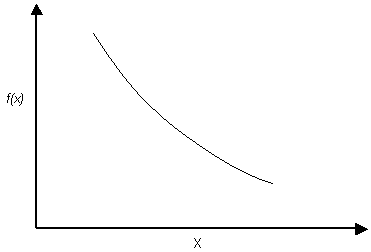Join one of our upcoming courses taking place around the world! Explore now

# Exponential Distribution

The Exponential probability density function is available in RocFall.

Exponential probability density functionNOTES:

• The range of values must always be positive for an Exponential distribution. It must therefore not be used for random variables which include negative values.
• The mean of an Exponential distribution is equal to its standard deviation. This is a property of the EXPONENTIAL distribution, and cannot be altered by the user. (This is why you cannot define a standard deviation for an Exponential distribution in RocFall).
• Like the Normal distribution, the Exponential distribution can be truncated by entering the desired minimum and maximum values (the basic Exponential distribution varies from zero to infinity).